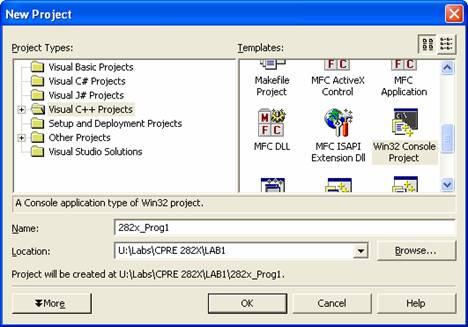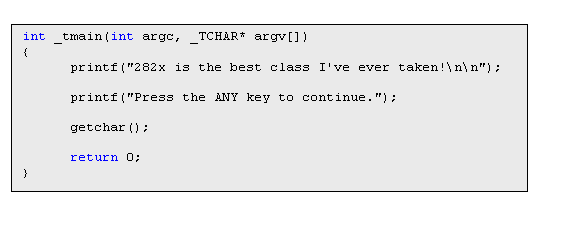Instructions to write C code in Visual Studio .NET 2003

1.   Start up Microsoft Visual Studio .NET. Visual Studio .Net will create a folder for each new project.  For 282x projects the recommended file path would be U:\Labs\CPRE282X\lab_number, (e.g. U:\Labs\CPRE282X\labw01b).

Start à All Programs à Microsoft Visual Studio .NET 2003

1. Start a new Project
1. File à New à Project…
2. In the ‘New Project’ window, under ‘Project Types:’ select ‘Visual C++ Projects’.  Then under Templates scroll down and select ‘Win32 Console Project’.  Then enter “282x_Prog1” as the ‘Name:’ and browse to the ‘Location:’ you want to save the project such as “U:\Labs\CPRE 282X\LAB1”.  If you need to setup a new file path you can do so at this time.  Below is a view of a completed window.
3. Next click on the ‘OK’ button.  Then on the next window click on the  ‘Finish’ button.4. Now you are looking at the main .cpp source file.  Although we are using Visual Studio C++ project, we can write C code in the main body and still compile and run it.

1. Next write the following C code in the main body:2. Run the program.
1. From the ‘Debug’ menu select ‘Start’.  A window will pop up asking if you want to build the project configuration(s).  Click on the ‘Yes’ button.

3. A terminal window will appear.  Follow your own instructions.  You have just created and executed a C program in Visual Studio .Net.  Run the program again to show your TA.

Program Difference Engine in C:

1.      You have learned difference engine in class. In this part of the lab, you are required to implement the difference engine in C. The skeleton of the program has been given in step 3.

2.      We are using sine function here. You can input DELTA value, which should be small, e.g. 0.01. x is set to 0 and x[i] = x[i-1] + Delta. Then we can compute y=sin(x), y=sin(x), y=sin(x), and y=sin(x). After we get y, y, y, and y, we can find the first column of the difference engine table, which are Delta0y, Delta1y, Delta2y, and Delta3y. Then, we can compute the remaining of the table. The x, y, Delta0y, Delta1y, Delta2y, and Delta3y arrays can be very large given enough memory. Therefore, we can look up the difference engine table and get the result of y=sin(x).

3.      Create a new Visual Studio .Net project for the difference engine problem. Copy and paste the following skeleton into the new project and complete the program. Output the first 10 columns of the table.

4.       Use your calculator to obtain the value of sin(x) for the x values from your table.  Verify that your difference engine has in fact calculated the sin.  Experiment by using different values for delta and checking the accuracy of the difference engine output compared to the actual value of sin.  Show your results to TA.

// 282xprog2.cpp : Defines the entry point for the console application.

//

// 282x_Prog2.cpp : Defines the entry point for the console application.

//

#include "stdafx.h"

//Difference Engine Program

#include <iostream>

#include "math.h"

#define column 100

int fact(int n)

{

if(n < 1)

return (1);

else

return (n*fact(n-1));

}

main()

{

int i = 0;

//Enter the value of DELTA

double Delta;

printf("Please enter the Delta value: ");

scanf("%lf", &Delta);

printf("Delta = %1.6lf \n", Delta);

//Calculate first several values: y0,y1,y2,y3,y4 given x0 and Delta

//compute x[i]

double x[column], y[column];

x = 0.00;

x = x + Delta;

x = x + Delta;

x = x + Delta;

x = x + Delta;

//compute sin(x[i])

y = x - pow(x,3)/fact(3) + pow(x,5)/fact(5);

y = x - pow(x,3)/fact(3) + pow(x,5)/fact(5);

y = x - pow(x,3)/fact(3) + pow(x,5)/fact(5);

y = x - pow(x,3)/fact(3) + pow(x,5)/fact(5);

//compute Delta0y[i], Delta1y[i], Delta2y[i], Delta3y[i]

//to get the first column

double Delta0y[column], Delta1y[column], Delta2y[column], Delta3y[column];

Delta0y = y ;

Delta0y = y ;

Delta0y = y ;

Delta0y = y ;

Delta1y = Delta0y-Delta0y;

Delta1y = Delta0y-Delta0y;

Delta1y = Delta0y-Delta0y;

Delta2y = Delta1y-Delta1y;

Delta2y = Delta1y-Delta1y;

//all the Delta3y[n] are the same

Delta3y = Delta2y-Delta2y;

//compute the first 10 columns

for (i=1; i<11; i++)

{

/*********************/

}

//print out the columns of Difference Machine Table

/*********************/

getchar();

getchar();

return(0);

}